# The Illustrated Self-Supervised Learning

I first got introduced to self-supervised learning in a talk by Yann Lecun, where he introduced the “cake analogy” to illustrate the importance of self-supervised learning. In the talk, he said:

“If intelligence is a cake, the bulk of the cake is self-supervised learning, the icing on the cake is supervised learning, and the cherry on the cake is reinforcement learning (RL).”

Though the analogy is debated, we have seen the impact of self-supervised learning in the Natural Language Processing field where recent developments (Word2Vec, Glove, ELMO, BERT) have embraced self-supervision and achieved state of the art results.Curious to know the current state of self-supervised learning in the Computer Vision field, I read up on existing literature on self-supervised learning applied to computer vision through a recent survey paper by Jing et. al.

In this post, I will explain what is self-supervised learning and summarize the patterns of problem formulation being used in self-supervised learning with visualizations.

## Why Self-Supervised Learning?

To apply supervised learning with deep neural networks, we need enough labeled data. To acquire that, human annotators manually label data which is both a time consuming and expensive process. There are also fields such as the medical field where getting enough data is a challenge itself. Thus, a major bottleneck in current supervised learning paradigm is the label generation part.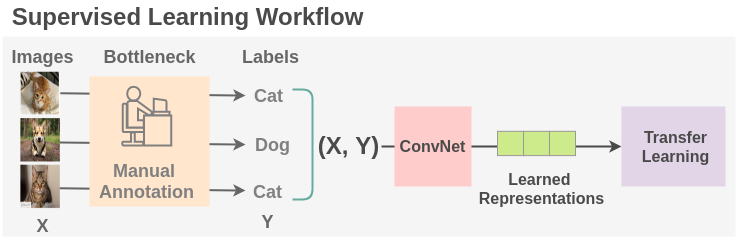## What is Self-Supervised Learning?

Self supervised learning is a method that poses the following question to formulate an unsupervised learning problem as a supervised one:

Can we design the task in such a way that we can generate virtually unlimited labels from our existing images and use that to learn the representations?In self-supervised learning, we replace the human annotation block by creatively exploiting some property of data to set up a pseudo-supervised task. For example, here instead of labeling images as cat/dog, we could instead rotate them by 0/90/180/270 degrees and train a model to predict rotation. We can generate virtually unlimited training data from millions of images we have freely available on the internet.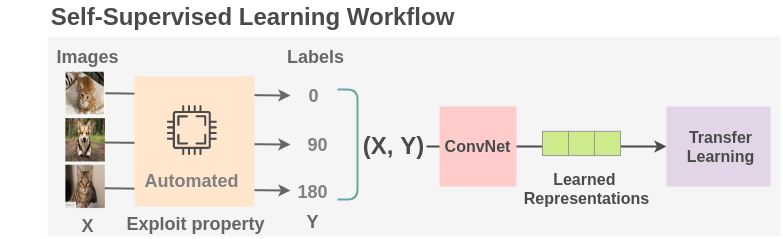Figure: End to End Workflow of Self-Supervised Learning

Once we learn representations from these millions of images, we can use transfer learning to fine-tune it on some supervised task like image classification of cats vs dogs with very few examples.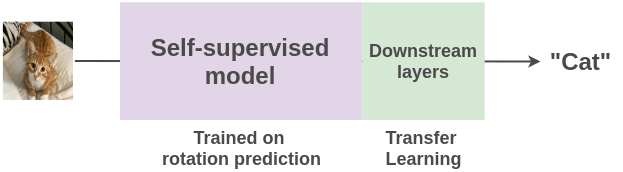## Survey of Self-Supervised Learning Methods

Let’s now understand the various approaches researchers have proposed to exploit image and video properties and apply self-supervised learning for representation learning.

## 1. Image Colorization

Formulation:

What if we prepared pairs of (grayscale, colorized) images by applying grayscale to millions of images we have freely available?We could use an encoder-decoder architecture based on a fully convolutional neural network and compute the L2 loss between the predicted and actual color images.To solve this task, the model has to learn about different objects present in the image and related parts so that it can paint those parts in the same color. Thus, representations learned are useful for downstream tasks.## 2. Image Superresolution

Formulation:

What if we prepared training pairs of (small, upscaled) images by downsampling millions of images we have freely available?GAN based models such as SRGAN are popular for this task. A generator takes a low-resolution image and outputs a high-resolution image using a fully convolutional network. The actual and generated images are compared using both mean-squared-error and content loss to imitate human-like quality comparison. A binary-classification discriminator takes an image and classifies whether it’s an actual high-resolution image(1) or a fake generated superresolution image(0). This interplay between the two models leads to generator learning to produce images with fine details.Both generator and discriminator learn semantic features that can be used for downstream tasks.

## 3. Image Inpainting

Formulation:

What if we prepared training pairs of (corrupted, fixed) images by randomly removing part of images?Similar to superresolution, we can leverage a GAN-based architecture where the Generator can learn to reconstruct the image while discriminator separates real and generated images.For downstream tasks, Pathak et al. have shown that semantic features learned by such a generator give 10.2% improvement over random initialization on the PASCAL VOC 2012 semantic segmentation challenge while giving <4% improvements over classification and object detection.

## 4. Cross-Channel Prediction

Formulation:

What if we predict one channel of the image from the other channel and combine them to reconstruct the original image?

Zhang et al. used this idea in their paper called “Split-Brain Autoencoder”. To understand the idea of the paper, let’s take an example of a color image of tomato.Example adapted from “Split-Brain Autoencoder” paper

For this color image, we can split it into grayscale and color channels. Then, for the grayscale channel, we predict the color channel and for the color channel part, we predict the grayscale channel. The two predicted channels $X_1$ and $X_2$ are combined to get back a reconstruction of the original image. We can compare this reconstruction to the original color image to get a loss and improve the model.

This same setup can be applied for images with depth as well where we use the color channels and the depth channels from a RGB-HHA image to predict each other and compare output image and original image.Example adapted from “Split-Brain Autoencoder” paper

## 1. Image Jigsaw Puzzle

Formulation:

What if we prepared training pairs of (shuffled, ordered) puzzles by randomly shuffling patches of images?Even with only 9 patches, there can be 362880 possible puzzles. To overcome this, only a subset of possible permutations is used such as 64 permutations with the highest hamming distance.Suppose we use a permutation that changes the image as shown below. Let’s use the permutation number 64 from our total available 64 permutations.Now, to recover back the original patches, Noroozi et al. proposed a neural network called context-free network (CFN) as shown below. Here, the individual patches are passed through the same siamese convolutional layers that have shared weights. Then, the features are combined in a fully-connected layer. In the output, the model has to predict which permutation was used from the 64 possible classes. If we know the permutation, we can solve the puzzle.To solve the Jigsaw puzzle, the model needs to learn to identify how parts are assembled in an object, relative positions of different parts of objects and shape of objects. Thus, the representations are useful for downstream tasks in classification and detection.

## 2. Context Prediction

Formulation:

What if we prepared training pairs of (image-patch, neighbor) by randomly taking an image patch and one of its neighbors around it from large, unlabeled image collection?To solve this pre-text task, Doersch et al. used an architecture similar to that of a jigsaw puzzle. We pass the patches through two siamese ConvNets to extract features, concatenate the features and do a classification over 8 classes denoting the 8 possible neighbor positions.## 3. Geometric Transformation Recognition

Formulation:

What if we prepared training pairs of (rotated-image, rotation-angle) by randomly rotating images by (0, 90, 180, 270) from large, unlabeled image collection?To solve this pre-text task, Gidaris et al. propose an architecture where a rotated image is passed through a ConvNet and the network has to classify it into 4 classes(0/90/270/360 degrees).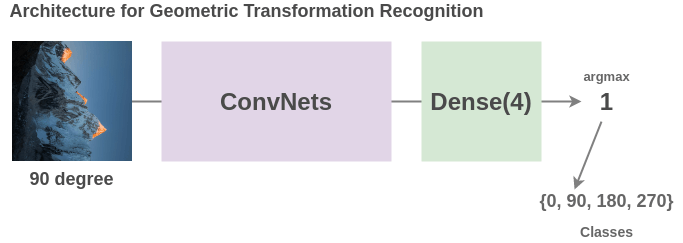Though a very simple idea, the model has to understand location, types and pose of objects in an image to solve this task and as such, the representations learned are useful for downstream tasks.

## 1. Image Clustering

Formulation:

What if we prepared training pairs of (image, cluster-number) by performing clustering on large, unlabeled image collection?To solve this pre-text task, Caron et al. propose an architecture called deep clustering. Here, the images are first clustered and the clusters are used as classes. The task of the ConvNet is to predict the cluster label for an input image.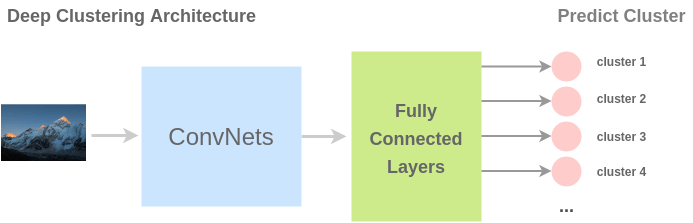Papers:

## 2. Synthetic Imagery

Formulation:

What if we prepared training pairs of (image, properties) by generating synthetic images using game engines and adapting it to real images?To solve this pre-text task, Ren et al. propose an architecture where weight-shared ConvNets are trained on both synthetic and real images and then a discriminator learns to classify whether ConvNet features fed to it is of a synthetic image or a real image. Due to adversarial nature, the shared representations between real and synthetic images get better.## 1. Frame Order Verification

Formulation:

What if we prepared training pairs of (video frames, correct/incorrect order) by shuffling frames from videos of objects in motion?To solve this pre-text task, Misra et al. propose an architecture where video frames are passed through weight-shared ConvNets and the model has to figure out whether the frames are in the correct order or not. In doing so, the model learns not just spatial features but also takes into account temporal features.Papers:

## Citation Info (BibTex)

If you found this blog post useful, please consider citing it as:

@misc{chaudhary2020selfsupervised,
title   = {The Illustrated Self-Supervised Learning},
author  = {Amit Chaudhary},
year    = 2020,
note    = {\url{https://amitness.com/2020/02/illustrated-self-supervised-learning}}
}